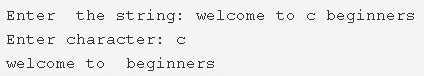# C Program To Remove Last Occurrence Of A Character From String

C program to remove the last occurrence of a character from a string – In this article, we will brief in on the various ways to remove the last occurrence of a character from a string in C programming.

Suitable examples and sample programs have also been added so that you can understand the whole thing very clearly. The compiler has also been added with which you can execute it yourself.

The ways used in this piece are as follows:

• Using Standard Method
• Using Function
• Using Recursion

A string is nothing but an array of characters. The value of a string is determined by the terminating character. Its value is considered to be 0.As we can see in the image uploaded above, we have to enter a string first.

The string entered here is as follows:

“welcome to c beginners”

Then we have to enter the character whose last occurrence we wanna delete.

The character is specified to be ‘c’.

Hence, the final string becomes

“welcome to   beginners”

Thus, means to do the same in C programming are as follows:

## Using Standard Method

1. Read user entered string using gets(s) function.

2) Read the entered character using getchar() and store in the variable c.

3) The first for loop iterates through the given string until the last character becomes to null with the structure for(i=0;s[i];i++).

4) Initialize n=i.i value represents the length of the string.

5) While loop iterates the code if ‘i’ value not equal to 0.

a) If temp=1 then decrease the i value and the inner if compares s[i] with the removable character. If it matches then shift the element which is right to the removed element, to one position left and assign temp=0.

b) If temp=0.

if i=n then break the loop.

if i!=n then shift the remaining elements which are right to the removed element to one position left as s[i]=s[i+1] and increase i value.

6) Print the string after removing the last occurrence of the element.

Output:

### Using Function

1. The main() calls the stringlength(char *s) to get the length of the string.

2) The main() calls the deletechar(char *s, char c) to remove the last occurrence of the element in the string.

Initialize temp=1 and i,n with the length of the string.

while loop iterates until i becomes to 0.

a) Checks the temp value if temp=1 then inner if compares the s[i] with the entered character. If it matches then initialize temp=0 and shift the element which is right to the removable element to one position left.

b) if temp=0

If  ‘i’ equal to n then break the loop. Otherwise, shift the remaining elements which are right to the removed element, to one position left by increasing the i value.

3) The main() prints the string which has the less string length than the original string.

Output:

### Using Recursion

1. The stringlength(char *s) returns the length of the string.

2) The main() function calls the recursive function deletechar(char *s,char c).

Initialize i=length of the string.

A) If the length of the string!=0

if temp=1

a) then decrease the i value.

b) If the element s[i] match with the entered character then initialize temp=0 and shift the right element to the removable element, to one position left and increase the i value.

B) If the length of the string is zero.

If i=n then break the loop. Otherwise, shift the remaining elements which are right to the removed element to one position left by increasing the i value.

Output:
x

## C Program To Check If Alphabet, Digit or Special Character | C Programs

C program to check for an alphabet, digit or special character – In this article, ...